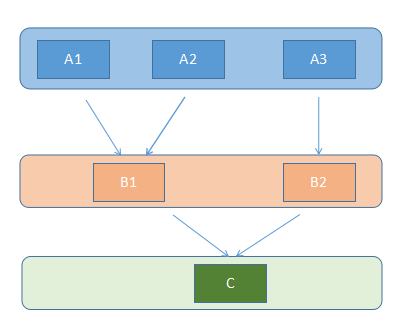# Python的MRO以及C3线性化算法

python3 中的方法解析顺序 (Method Resolution Order , MRO)采用C3线性化算法来确定

(百度Python MRO排在首位的文章，绝大部分内容是正确的，但是核心公式错了)

L[object] = [object]
L[C(B1…BN)] = [C] + merge(L[B1]…L[BN], [B1, … ,BN])

1. 检查第一个列表的头元素（如 L[B1] 的头），记作 H。
2. 若 H 未出现在其它列表的尾部，则将其输出，并将其从所有列表中删除，然后回到步骤1；否则，取出下一个列表的头部记作 H，继续该步骤。
3. 重复上述步骤，直至列表为空或者不能再找出可以输出的元素。如果是前一种情况，则算法结束；如果是后一种情况，说明无法构建继承关系，Python 会抛出异常。L[B1(A1,A2)] = [B1] + merge(L[A1], L(A2), [A1, A2])
= [B1] + merge([A1,Obj], [A2,Obj], [A1,A2])
= [B1, A1] + merge([Obj], [A2,Obj], [A2])
= [B1, A1, A2] + merge([Obj], [Obj])
= [B1, A1, A2, Obj]

L[B2(A3)] = [B2, A3, Obj]

L[C(B1,B2)] = [C] + merge(L[B1(A1,A2)], L[B2(A3)], [B1,B2])
= [C] + merge([B1, A1, A2, Obj], [B2, A3, Obj], [B1,B2])
= [C, B1] + merge([A1, A2, Obj], [B2, A3, Obj], [B2])
= [C, B1, A1] + merge([A2, Obj], [B2, A3, Obj], [B2])
= [C, B1, A1, A2] + merge([Obj], [B2, A3, Obj], [B2])
= [C, B1, A1, A2, B2] +merge([Obj], [A3, Obj])
= [C, B1, A1, A2, B2, A3] +merge([Obj], [Obj])
= [C, B1, A1, A2, B2, A3, Obj]

C.mro()

[<class '__main__.C'>, <class '__main__.B1'>, <class '__main__.A1'>, <class '__main__.A2'>, <class '__main__.B2'>, <class '__main__.A3'>, <class 'object'>]



posted @ 2019-05-25 16:26  喵帕喵帕喵  阅读(820)  评论(0编辑  收藏  举报